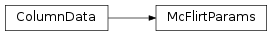# mvpa2.misc.fsl.base.McFlirtParams¶class `mvpa2.misc.fsl.base.``McFlirtParams`(source)

Read and write McFlirt’s motion estimation parameters from and to text files.

Attributes

 `ncolumns` Returns the number of columns. `nrows` Returns the number of rows.

Methods

 `clear`(() -> None.  Remove all items from D.) `copy`(() -> a shallow copy of D) `fromkeys`(...) v defaults to None. `get`((k[,d]) -> D[k] if k in D, ...) `has_key`((k) -> True if D has a key k, else False) `items`(() -> list of D’s (key, value) pairs, ...) `iteritems`(() -> an iterator over the (key, ...) `iterkeys`(() -> an iterator over the keys of D) `itervalues`(...) `keys`(() -> list of D’s keys) `plot`() Produce a simple plot of the estimated translation and rotation parameters using. `pop`((k[,d]) -> v, ...) If key is not found, d is returned if given, otherwise KeyError is raised `popitem`(() -> (k, v), ...) 2-tuple; but raise KeyError if D is empty. `select_samples`(selection) Return new ColumnData with selected samples `setdefault`((k[,d]) -> D.get(k,d), ...) `toarray`() Returns the data as an array with six columns (same order as in file). `tofile`(filename) Write motion parameters to file. `update`(([E, ...) If E present and has a .keys() method, does: for k in E: D[k] = E[k] `values`(() -> list of D’s values) `viewitems`(...) `viewkeys`(...) `viewvalues`(...)

Initialize McFlirtParams

Parameters: source : str Filename of a parameter file.

Attributes

 `ncolumns` Returns the number of columns. `nrows` Returns the number of rows.

Methods

 `clear`(() -> None.  Remove all items from D.) `copy`(() -> a shallow copy of D) `fromkeys`(...) v defaults to None. `get`((k[,d]) -> D[k] if k in D, ...) `has_key`((k) -> True if D has a key k, else False) `items`(() -> list of D’s (key, value) pairs, ...) `iteritems`(() -> an iterator over the (key, ...) `iterkeys`(() -> an iterator over the keys of D) `itervalues`(...) `keys`(() -> list of D’s keys) `plot`() Produce a simple plot of the estimated translation and rotation parameters using. `pop`((k[,d]) -> v, ...) If key is not found, d is returned if given, otherwise KeyError is raised `popitem`(() -> (k, v), ...) 2-tuple; but raise KeyError if D is empty. `select_samples`(selection) Return new ColumnData with selected samples `setdefault`((k[,d]) -> D.get(k,d), ...) `toarray`() Returns the data as an array with six columns (same order as in file). `tofile`(filename) Write motion parameters to file. `update`(([E, ...) If E present and has a .keys() method, does: for k in E: D[k] = E[k] `values`(() -> list of D’s values) `viewitems`(...) `viewkeys`(...) `viewvalues`(...)
`header_def` = ['rot1', 'rot2', 'rot3', 'x', 'y', 'z']
`plot`()

Produce a simple plot of the estimated translation and rotation parameters using.

You still need to can pylab.show() or pylab.savefig() if you want to see/get anything.

`toarray`()

Returns the data as an array with six columns (same order as in file).

`tofile`(filename)

Write motion parameters to file.